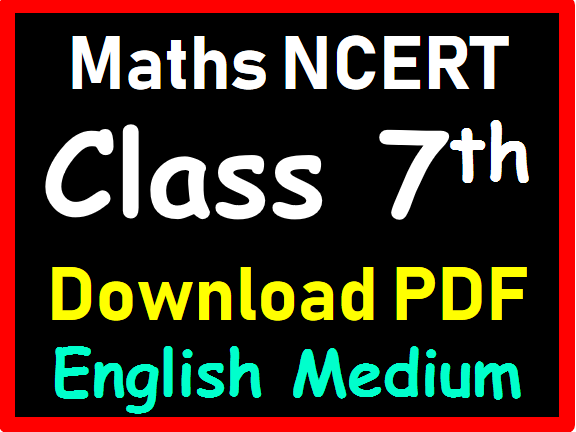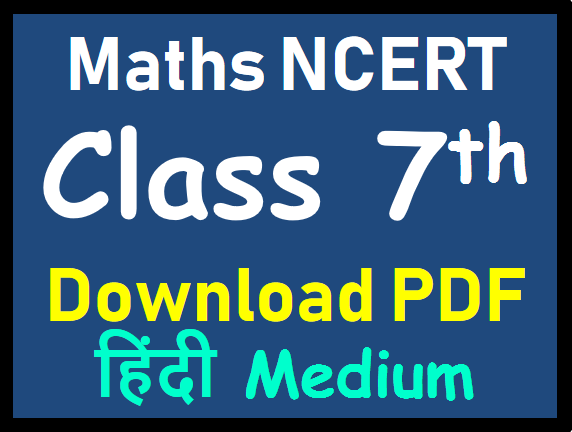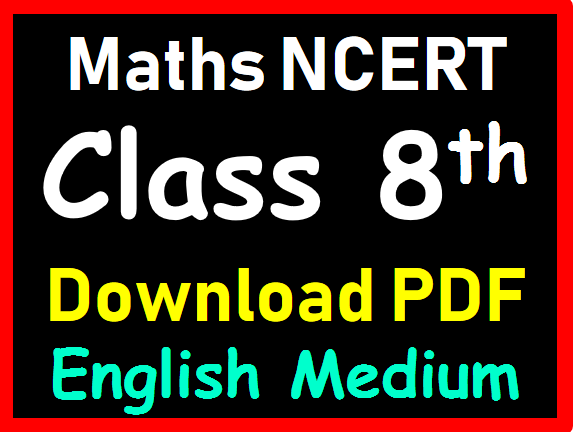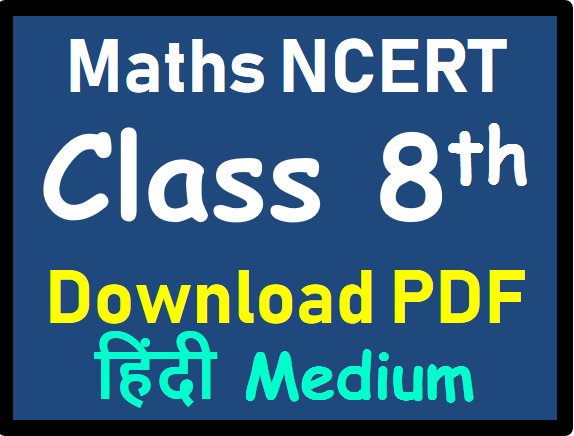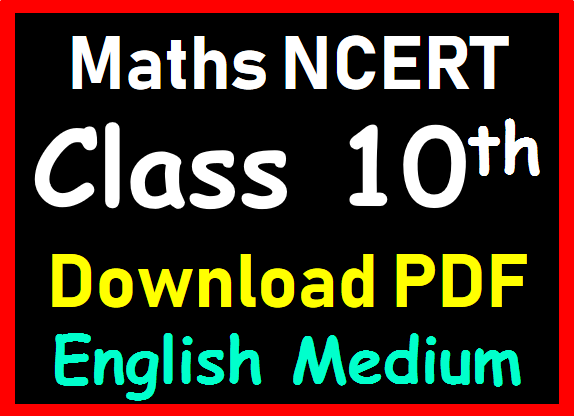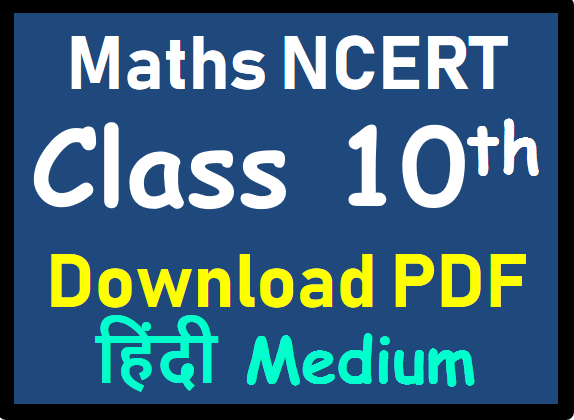# Importance of NCERT for SSC CGL Maths Preparation

NCERT books are considered to be the most important books if you are studying for board exams. The students must ensure that all the contents in the books are properly understood. The concepts in the books have been very carefully and nicely explained in self-explanatory manner which are easy to learn and understand.

NCERT is sufficient source to complete Maths portion of SSC CGL CHSL or any other SSC Exam. Every SSC Aspirant who is preparing for SSC CGL or CHSL Exam must refer NCERTs at least once to cover all the concepts of Advance Maths. Here you will ALL PDFs of Maths NCERTs.

There are some key benefits of studying NCERT books while preparing for SSC Exams and NCERT Exemplar Problems, for SSC Aspirants are stated below:
• Provide in-depth knowledge in easy language. …
• Sufficient Source, no need to buy any other material. …
• Clears all fundamental concepts. …
• Best for the SSC and Railway exams. …
• Offers a number of basic problems to practice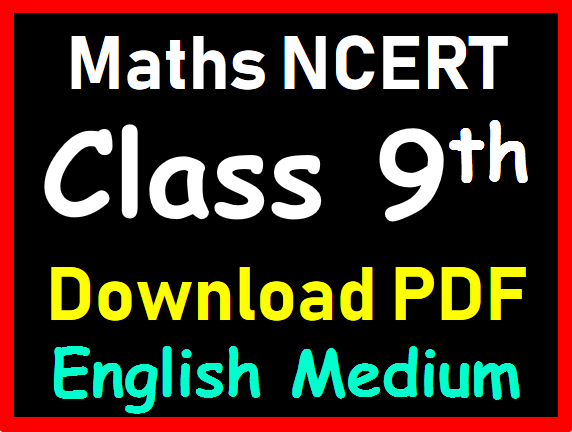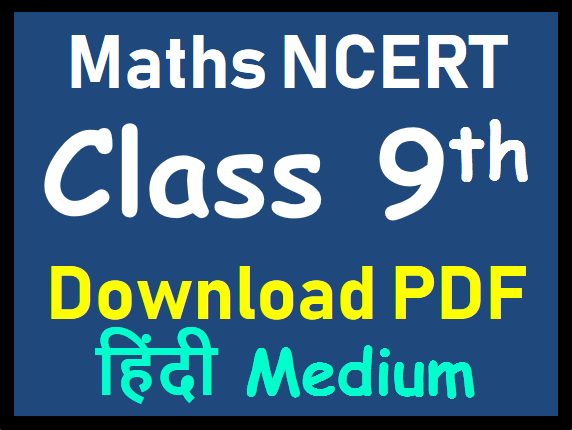## Class 9th Maths NCERT

COMPLETE SYLLABUS (Contents)

1. NUMBER SYSTEMS 1
1.1 Introduction 1
1.2 Irrational Numbers 5
1.3 Real Numbers and their Decimal Expansions 8
1.4 Representing Real Numbers on the Number Line 15
1.5 Operations on Real Numbers 18
1.6 Laws of Exponents for Real Numbers 24
1.7 Summary 27

2. POLYNOMIALS 28
2.1 Introduction 28
2.2 Polynomials in One Variable 28
2.3 Zeroes of a Polynomial 32
2.4 Remainder Theorem 35
2.5 Factorisation of Polynomials 40
2.6 Algebraic Identities 44
2.7 Summary 50

3. COORDINATE GEOMETRY 51
3.1 Introduction 51
3.2 Cartesian System 54
3.3 Plotting a Point in the Plane if its Coordinates are given 61
3.4 Summary 65

4. LINEAR EQUATIONS IN TWO VARIABLES 66
4.1 Introduction 66
4.2 Linear Equations 66
4.3 Solution of a Linear Equation 68
4.4 Graph of a Linear Equation in Two Variables 70
4.5 Equations of Lines Parallel to x-axis and y-axis 75
4.6 Summary 77

5. INTRODUCTION TO EUCLID’S GEOMETRY 78
5.1 Introduction 78
5.2 Euclid’s Definitions, Axioms and Postulates 80
5.3 Equivalent Versions of Euclid’s Fifth Postulate 86
5.4 Summary 88

6. LINES AND ANGLES 89
6.1 Introduction 89
6.2 Basic Terms and Definitions 90
6.3 Intersecting Lines and Non-intersecting Lines 92
6.4 Pairs of Angles 92
6.5 Parallel Lines and a Transversal 98
6.6 Lines Parallel to the same Line 101
6.7 Angle Sum Property of a Triangle 105
6.8 Summary 108

7. TRIANGLES 109
7.1 Introduction 109
7.2 Congruence of Triangles 109
7.3 Criteria for Congruence of Triangles 112
7.4 Some Properties of a Triangle 120
7.5 Some More Criteria for Congruence of Triangles 125
7.6 Inequalities in a Triangle 129
7.7 Summary 134

8.1 Introduction 135
8.2 Angle Sum Property of a Quadrilateral 136
8.4 Properties of a Parallelogram 139
8.5 Another Condition for a Quadrilateral to be a Parallelogram 145
8.6 The Mid-point Theorem 148
8.7 Summary 151

9. AREAS OF PARALLELOGRAMS AND TRIANGLES 152
9.1 Introduction 152
9.2 Figures on the same Base and Between the same Parallels 154

9.3 Parallelograms on the same Base and
between the same Parallels 156
9.4 Triangles on the same Base and between
the same Parallels 160
9.5 Summary 167

10. CIRCLES 168
10.1 Introduction 168
10.2 Circles and its Related Terms : A Review 169
10.3 Angle Subtended by a Chord at a Point 171
10.4 Perpendicular from the Centre to a Chord 173
10.5 Circle through Three Points 174
10.6 Equal Chords and their Distances from the Centre 176
10.7 Angle Subtended by an Arc of a Circle 179
10.9 Summary 187

11. CONSTRUCTIONS 187
11.1 Introduction 188
11.2 Basic Constructions 189
11.3 Some Constructions of Triangles 191
11.4 Summary 196

12. HERON’S FORMULA 197
12.1 Introduction 197
12.2 Area of a Triangle – by Heron’s Formula 199
12.3 Application of Heron’s Formula in finding
12.4 Summary 207

13. SURFACE AREAS AND VOLUMES 208
13.1 Introduction 208
13.2 Surface Area of a Cuboid and a Cube 208
13.3 Surface Area of a Right Circular Cylinder 214
13.4 Surface Area of a Right Circular Cone 217
13.5 Surface Area of a Sphere 222
13.6 Volume of a Cuboid 226
13.7 Volume of a Cylinder 228
13.8 Volume of a Right Circular Cone 231
13.9 Volume of a Sphere 234
10.10 Summary 237

14. STATISTICS 238
14.1 Introduction 238
14.2 Collection of Data 239
14.3 Presentation of Data 240
14.4 Ggraphical Representation of Data 247
14.5 Measures of Central Tendency 261
14.6 Summary 270

15. PROBABILITY 271
15.1 Introduction 271
15.2 Probability – an Experimental Approach 272
15.3 Summary 285

# Maths NCERTs from Class 6th to 10th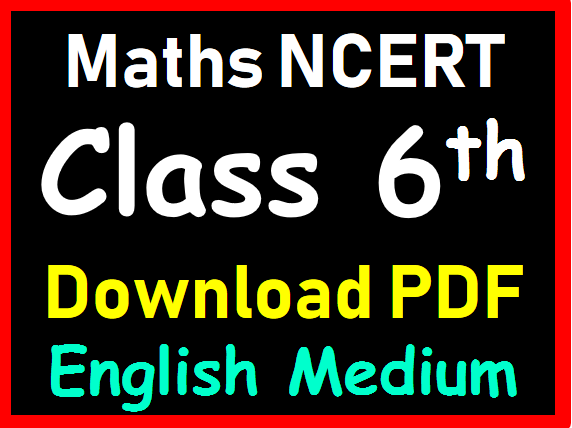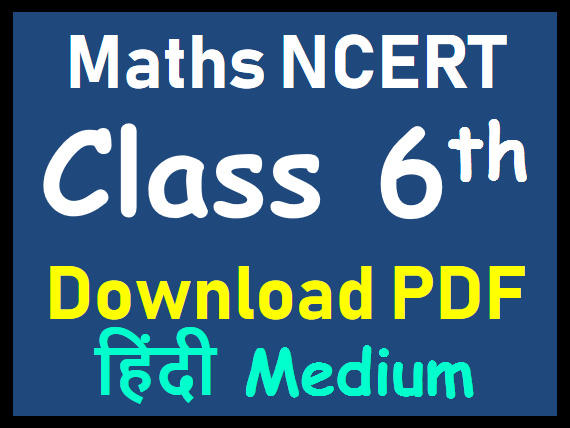##